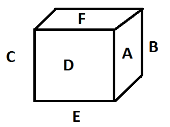# Reasoning - Symbol Sequence

A dice is a small cube and a number of dots, letters, numbers, or figures can be drawn on the six faces of dice. Dice problems are frequently asked in candidate selection process. Six faces of a dice can be made by folding a piece of paper cut in a predetermined shape having six squares as described in the figure.

Let’s have a piece of paper such as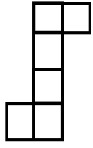which can be folded to form a cube in following manner.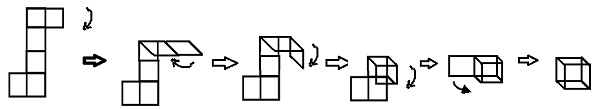Various faces of a dice have been described in the figures.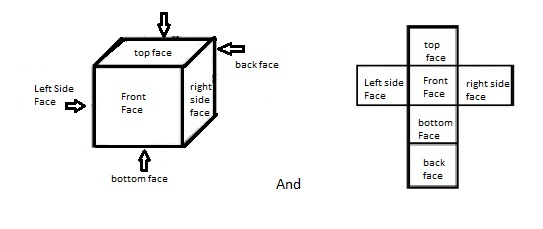In competitive exams, candidates are asked to solve various questions based on different portion of dice in picture form such as to find opposite faces, bottom face etc. Question may also be based on an unfolded figure of piece of cut in a shape that can be folded to form a cube/dice.

To find opposite faces, candidate may get various figures of dice showing two, three or four positions of a dice. Candidate has to compare various figures, numbers, or letters given on six faces of dice to get the answer.

Before going to solve problem on dice, a candidate should master himself to analyze the figure and locate exact position of figures, letter, or numbers on six faces of dice. There are two relations basically involved between two faces of dice either they are opposite to each other like front and back side or adjacent to each other. Example given below can explain this issue clearly.

Suppose on six faces of a dice numbers from 1 to 6 have been written.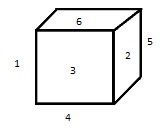In the figure, various opposite faces are 1-2, 3-5 and 4-6 also 1, 2, 4 and 6 are adjacent to 3 and 5. It should be noted that opposite faces are always adjacent to same set of faces like 3 and 5 in the given figure.

Questions are mainly asked to find opposite faces which can be solved by equating the letters, figures or numbers adjacent to asked question letters, figures or numbers. The letters, figures or numbers left after equation would be opposite face. Questions can also be solved by arranging letters, figures or numbers on unfolded figure of dice as discussed in various cases.

### Case-1

When two positions of dice are given

Example

Two positions of a dice have been given whose faces are numbered from 1 to 6.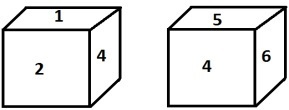Which number is opposite to 3?

Options

A - 4

B - 5

C - 6

D - 2

Explanation − In the given figures 4 is common to both positions of dice from which it is clear that adjacent face of 4 are 1, 2, 5,and 6. Hence number 3 is left after equating adjacent faces of 4 therefore it must be on opposite face of 4 and vice versa.

### Case-2

When three vies of a dice is given.

In this case, questions may be asked to find out opposite face or in given question figure there will be some missing character in any one view of dice and candidate has to compare various letters, figures, or numbers as per given question figure to find out answer letter, figure, or number.

Example-1

Following are three position of a dice given in the figures.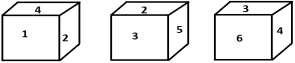Which number is opposite to 6?

Options

A - 1

B - 3

C - 4

D - 2

Explanation − It can be seen from the figures (i) and (ii) that 1, 3, 4 and 5 are adjacent to 2 hence only 6 must be opposite to 2 and vice-versa. It can also be solved by drawing unfold figure of dice accordingly with the question figure as,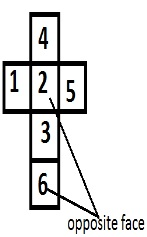Question may also ask to find missing letter, figure or number. Let us see this in the following example.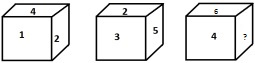Which number will replace question mark?

Options

A - 3

B - 4

C - 5

D - 6

Explanation − From the unfold figure, it is clear that 1, 4, 3 and 5 will be adjacent to 2 indicating 6 as on its opposite face. Unfold figure of dice can be drawn as follows.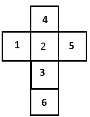### Case-3

In some exams, candidate may be provided with four position of dice, various questions may be asked related to the given figure are,

• Bottom face or back face of any of the position of dice given in the question figure.

• Choosing certain statement correct or incorrect based on the question figure.

• To find out pair of opposite faces.

For an example consider following positions of a dice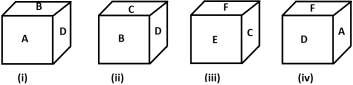1 - Which letter is on the bottom face of the figure (iii)?

Options

A - A

B - B

C - C

D - D

Explanation − We can draw unfold figure from figure (i) and (ii) A, C, and D will lie adjacent to B also from figure (i), (ii), and (iii) A, B, C and F are adjacent to D, therefore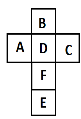2 - Which statement is true?

Options

A - A and C are opposite faces

B - At the back face of figure (iii) is D

C - Both are true

D - None

Explanation − From the unfold figure it is clear that option (c) is the answer.### Case-4

Sometimes the unfold figure of dice may be given and candidate has to answer various questions based on figure which may be to choose similar dice from alternative given or opposite face may also be asked to find. This type of problems can be solved by drawing folded figure of dice as explained by example here, let us have the following unfolded figure of a dice.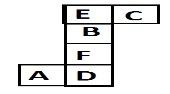Which dice is similar to the question figure?